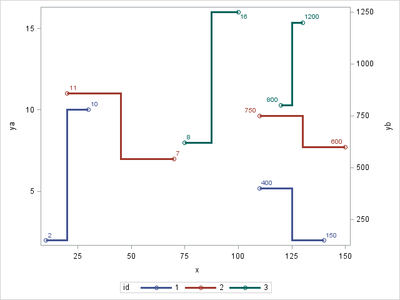## proc sgplot 2 axes

Hello all,

I have this dataset:

id    group   x           y

1     A          10        2

1     A          30       10

1     B          50      400

1     A         100      15

2     B         110      800

2     A          20       11

2     B         150      600

2     A          90        7

3     A         130       10

3     A          75        8

3     B          60       1200

3     B          50       1400

What I want to get is two curves in the same graph (using sgplot) for every id. The group A will follow xaxis and yaxis and the group B will follow xaxis and y2axis.  I didn't get the solution using this code (I should change it a bit):

proc sgplot data=tab1;
by id;
title "#byval1" justify=center ;
step x=x y=y / justify=center group=group lineattrs=(pattern=solid thickness=0.8mm) ;
xaxis minor minorcount=4 offsetmin=0 offsetmax=0 type=linear values=(0 to 110 by 10) ;
yaxis minor minorcount=4 offsetmin=0 offsetmax=0 type=linear values=(0 to 15 by 1) ;
y2axis minor minorcount=4 offsetmin=0 offsetmax=0 type=linear values=(0 to 1600 by 200) ;
run;

1 ACCEPTED SOLUTION

Accepted Solutions

## Re: proc sgplot 2 axes

Right now, you are plotting 2 lines for each STEP statement because of your group= option.

Drop the GROUP= option in both of your statements because you handle these groups manually by splitting up y 🙂

4 REPLIES 4

## Re: proc sgplot 2 axes

split your y variable up in two different variables and spefify two distinct step statements, one for each new y variable and use the y2axis option in one of the step statements 🙂

## Re: proc sgplot 2 axes

I did but it doesn't work. Following your suggestion, it will read only the first step statement. I tried something different which is the following:

proc sgplot data=tab1;

.

.

.

step x=x y=y / justify=center group=group lineattrs=(color=grey pattern=solid thickness=1.2mm) ;
step x=x y=y / y2axis justify=center group=extrt lineattrs=(color=green pattern=solid thickness=1.2mm) ;

.

.

run;

But I got 4 curves and not 2.

## Re: proc sgplot 2 axes

Right now, you are plotting 2 lines for each STEP statement because of your group= option.

Drop the GROUP= option in both of your statements because you handle these groups manually by splitting up y 🙂

## Re: proc sgplot 2 axes

Are you looking for something like this?``````data plotdata;
infile cards dlm=",";
input
id group \$ x y
;

if group = "A" then do;
ya = y;
end;

if group = "B" then do;
yb = y;
end;
cards;
1,A,10,2
1,A,30,10
1,B,110,400
1,B,140,150
2,B,110,750
2,A,20,11
2,B,150,600
2,A,70,7
3,A,100,16
3,A,75,8
3,B,120,800
3,B,130,1200
;

proc sort data=plotdata;
by x group id ;
run;

proc sgplot data=plotdata nocycleattrs;
step x=x y=ya /
justify=center group=id markers lineattrs=(pattern=solid thickness=0.8mm)
datalabel
;
step x=x y=yb /
justify=center group=id markers lineattrs=(pattern=solid thickness=0.8mm)
datalabel
y2axis
;
``````

Discussion stats
• 4 replies
• 1168 views
• 0 likes
• 3 in conversation